International
Tables for
Crystallography
Volume C
Mathematical, physical and chemical tables
Edited by E. Prince

International Tables for Crystallography (2006). Vol. C, ch. 6.4, p. 611

## Section 6.4.9. Secondary extinction

T. M. Sabinea

aANSTO, Private Mail Bag 1, Menai, NSW 2234, Australia

### 6.4.9. Secondary extinction

| top | pdf |

A separate treatment of secondary extinction is required only in the uncorrelated block model, and the method given by Hamilton (1957) is used in this work. The coupling constant in the H–D equations is given by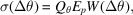where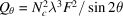for equatorial reflections in the neutron case,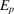is the correction for primary extinction evaluated at the angle θ, and W(Δθ) is the distribution function for the tilts between mosaic blocks. The choice of this function has a significant influence on the final result (Sabine, 1985), and a rectangular or triangular form is suggested.

In the following equations for the secondary-extinction factor,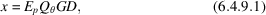and A and B are given by equations (6.4.5.6)and (6.4.5.7). The average path length through the crystal for the reflection under consideration is D and G is the integral breadth of the angular distribution of mosaic blocks. It is important to note that A should be set equal to one if the data have been corrected for absorption, and B should be set equal to one if absorption-weighted values of D are used. If D for each reflection is not known, the average dimension of the crystal may be used for all reflections.

For a rectangular function, W(Δθ) = G for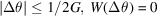otherwise, and the secondary-extinction factor becomes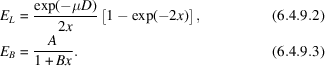For a triangular function, W(Δθ) =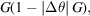for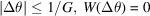otherwise, and the secondary-extinction factor becomes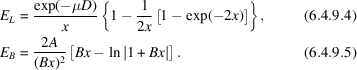### References

Hamilton, W. C. (1957). The effect of crystal shape and setting on secondary extinction. Acta Cryst. 10, 629–634.
Sabine, T. M. (1985). Extinction in polycrystalline materials. Aust. J. Phys. 38, 507–518.